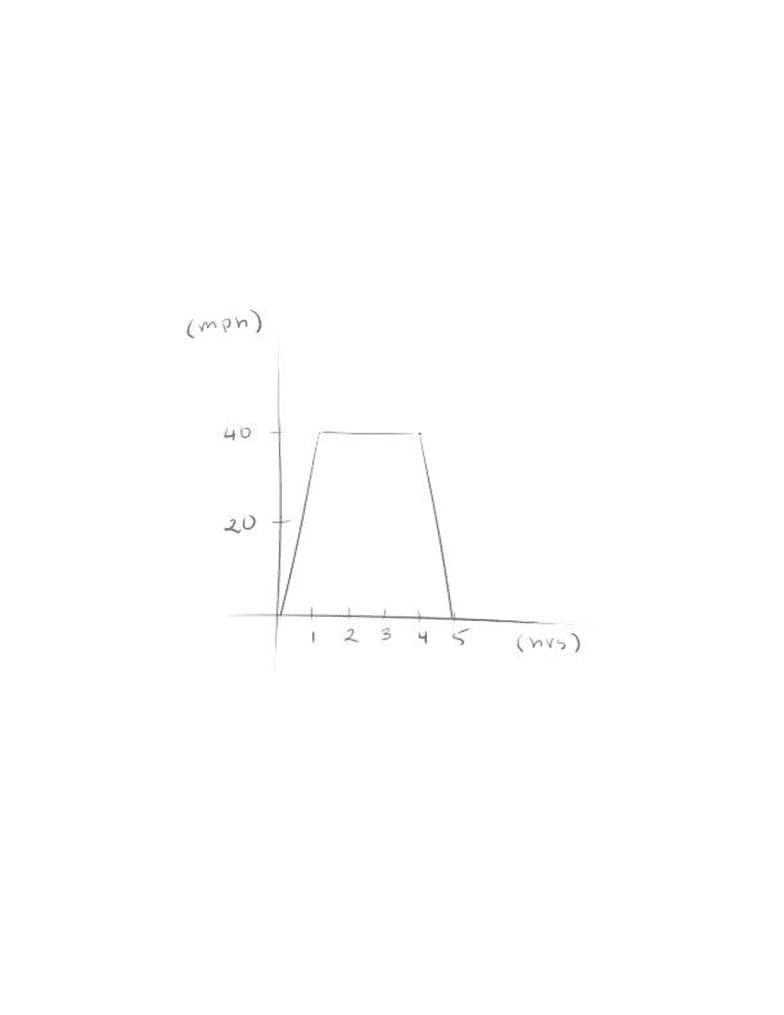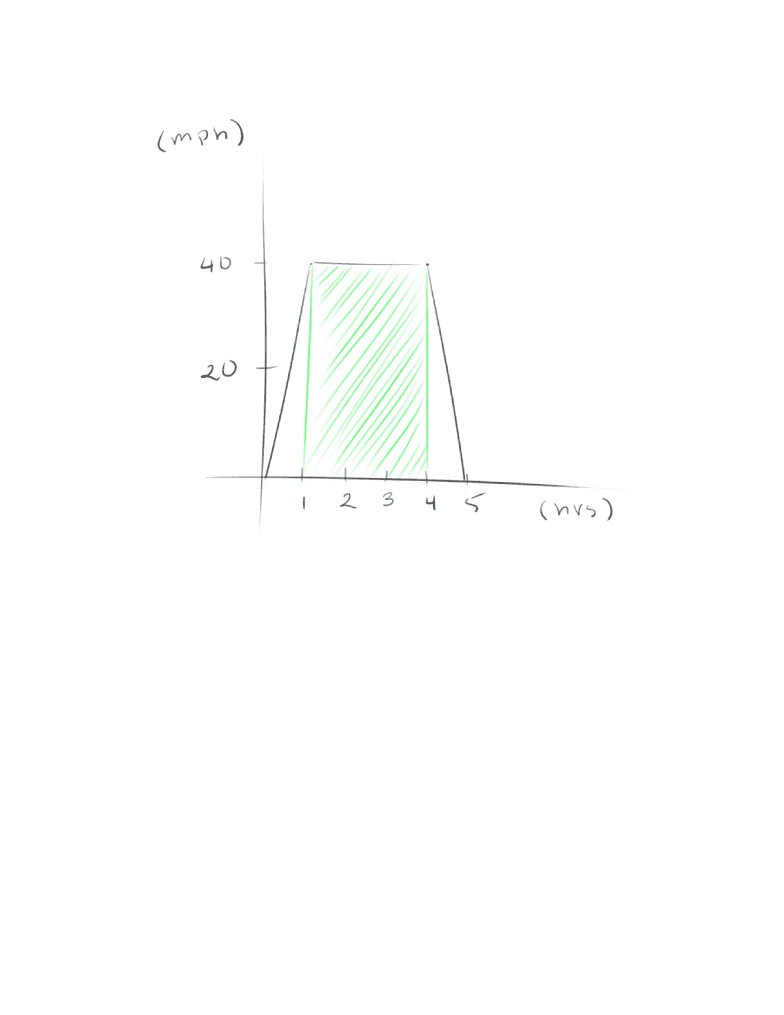Study Guides (390,000)
US (220,000)
UC-Irvine (3,000)
MATH (600)
MATH 2B (100)
All (60)
Midterm

# MATH 2B Study Guide - Midterm Guide: Formula DPremium

Department
Mathematics
Course Code
MATH 2B
Professor
All
Study Guide
Midterm

This preview shows pages 1-2. to view the full 6 pages of the document.Given the graph, what is the distance travelled
Between 1 and 4 pm?
In total? (from t = 0 to t = 5)
So we know that the graph shows the relationship between velocity (miles per hour)
and time (hours)
Because D (distance) is equal to the product of R (rate) and T (time), we can find
and solve for distance using the formula D = RT
Using the givens, between 1 and 4 pm is 3 hours, and the speed is constant at 40
mph
So D = (40)(3) = 120 miles
Looking at the graph, you may realize that 120 is equal to the area of the rectangle
formed using the time as a base and velocity as a height
Areas and Distances
Section 5.1
Typically seen questions

Only pages 1-2 are available for preview. Some parts have been intentionally blurred.

Subscribers OnlyThis is a fact that within a velocity time graph, the area under the curve is the
distance travelled
Knowing this information, we can then solve for the distance by finding the area
under the curve between t = 0 and t = 5
We can either break this into two triangles and a rectangle, or simply use the equation
of Area for a trapezoid
(½)(b1 + b2)(h) in which b1 is equal to base 1, b2 is base 2, and h is the height
(½)(3 + 5)(40) = 160 miles
Area Under the Curve Between Interval [a, b] (Theoretical)
Estimating area using rectangles
So say we have the function y = x2
We want to find the area under the curve between [0, 3]